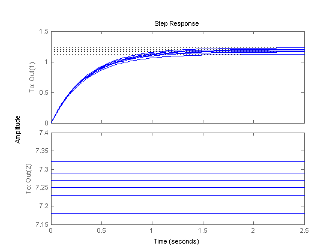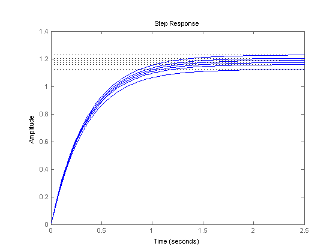## Select Models from Array

This example shows how to select individual models or sets of models from a model array using array indexing.

1. Load the transfer function array `m2d` into the MATLAB® workspace.

```load LTIexamples m2d ```
2. (Optional) Plot the step response of `m2d`.

`step(m2d)`The step response shows that `m2d` contains six one-input, two-output models. The `step` command plots all of the models in an array on a single plot.

3. (Optional) Examine the dimensions of `m2d`.

```arraydim = size(m2d) ```

This command produces the result:

```arraydim = 2 1 2 3```
• The first entries of `arraydim`, 2 and 1, show that `m2d` is an array of two-output, one-input transfer functions.

• The remaining entries in `arraydim` give the array dimensions of `m2d`, 2-by-3.

In general, the dimensions of a model array are `[Ny,Nu,S1,...,Sk]`. `Ny` and `Nu` are the numbers of outputs and inputs of each model in the array. `S1,...,Sk` are the array dimensions. Thus, `Si` is the number of models along the ith array dimension.

4. Select the transfer function in the second row, first column of `m2d`.

To do so, use MATLAB array indexing.

`sys = m2d(:,:,2,1)`

Tip

You can also access models using single index referencing of the array dimensions. For example,

`sys = m2d(:,:,4)`
selects the same model as `m2d(:,:,2,1)`.

5. Select the array of subsystems from the first input to the first output of each model in `m2d`.

`m11 = m2d(1,1,:,:)`
6. (Optional) Plot the step response of `m11`.

`step(m11)`The step response shows that `m11` is an array of six single-input, single-output (SISO) models.

Note

For frequency response data (FRD) models, the array indices can be followed by the keyword `'frequency'` and some expression selecting a subset of the frequency points, as in:

```sys(outputs,inputs,n1,...,nk,'frequency',SelectedFreqs) ```